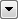You are here: Calculated Values > MeasureGenerator

# MeasureGenerator

Creates a set of measures attached to the geometry of the feature, where each value is the distance from the start of the line up to that vertex, multiplied by the given Multiplier.

This transformer is often used to load useful measure values into an attribute for writing to measure-supporting formats such as Shape, Geodatabase, and SDE.

## Related Transformers

• To get measures as a list or a series of attributes (for example, if you need to manipulate the calculated measure values), place a MeasureGenerator followed by a MeasureExtractor and a ListConcatenator.
• To calculate the entire length of a feature, use the LengthCalculator.
• To calculate the partial length of a feature, use the LengthToPointCalculator.

## Editing Transformer Parameters

Using a set of menu options, transformer parameters can be assigned by referencing other elements in the workspace. More advanced functions, such as an advanced editor and an arithmetic editor, are also available in some transformers. To access a menu of these options, clickbeside the applicable parameter. For more information, see Transformer Parameter Menu Options.